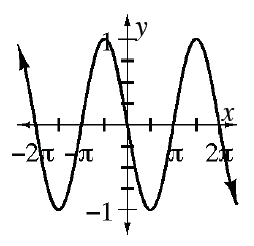### Home > INT3 > Chapter Ch12 > Lesson 12.2.3 > Problem12-111

12-111.

Consider the function $f(x)=\cos(x+\frac{\pi}{2})$.

1. Make a complete graph of the function.2. Write a simpler trigonometric function that is represented by the same graph.

Which trig function passes through the origin?

3. Now use the angle sum identity to expand and simplify $\cos(x+\frac{\pi}{2})$.

Review the Math Notes box in this lesson and problem 12-108 for the Angle Sum Identities.

4. Does your work in part (c) agree with your graphical results from parts (a) and (b)? Explain.